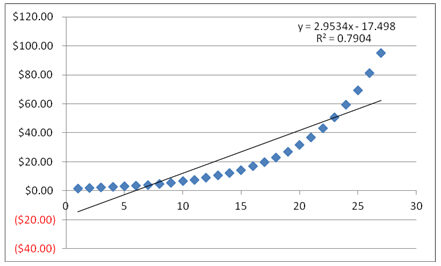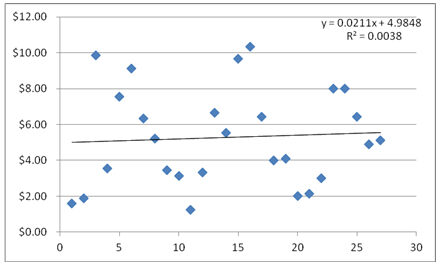## What is Goodness of Fit?

Goodness of fit (also known as a chi-square goodness of fit) is a statistical term referring to how far apart the expected values of a financial model are from the actual values.

## Goodness of Fit Example

Goodness of fit is a component of regression analysis, which is a statistical method used in finance and a variety of other fields to make predictions based on observed values. In other words, it is a measure of how correlated a group of actual observations are to a model's predictions.

In the following examples, the blue dots represent actual observations. The black lines represent a regression line, which is represented by the formula in the top right-hand corner of each chart. This formula is what analysts use to predict future values based on the behavior of the actual observations.

As you can see, this regression line has a high goodness of fit as represented by a high R2 value of 79%:This next chart is an example of low goodness of fit. Here, the formula for the regression line was virtually unable to come up with the observed values. Goodness of fit, as represented by R2, is only 0.38%:## Why Goodness of Fit Matters

Goodness of fit isn't just a statistical measure, it's a confidence measure. This is because when you've come up with a formula that accounts for most of the variations in a group of, say, price observations, you've also come up with a formula that can be a very reliable predictor of what prices will be in the future.